# Newton's Second Law Of Motion with Examples

Newton’s Second Law of Motion: In the previous topics I said that force causes acceleration. Moreover, we also learned the net force concept in the last section. Now, we deal with the relation between force and acceleration. As you remember, acceleration is the rate of change in the velocity of the object. This change occurs because of the net force. Thus, we can say that there is a linear relation between the net force acting on the object and acceleration. We show this relation like;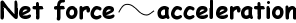If we increase the amount of net force than the acceleration also increases in the same amount. If we decrease the net force than acceleration also decreases. Let’s see it from the given picture below.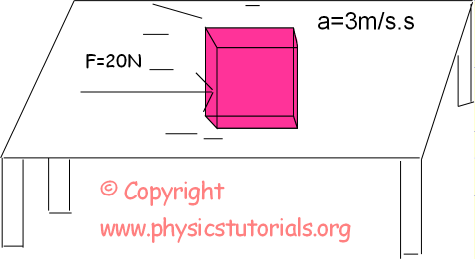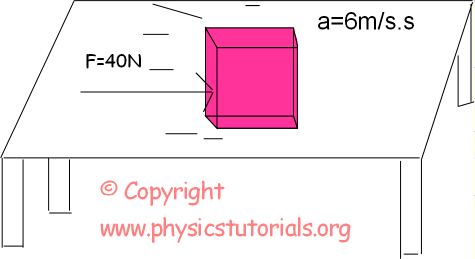In the Picture given above we double the force than the magnitude of acceleration also becomes double. On the contrary we decrease the force then, acceleration also decreases. We understand the relation between force and acceleration. Well, do you think mass affects the acceleration? Suppose that you push a box that is empty, you can easily push it. If the box is full, then can you push it easily with the same force? The answer is of course “NO”. All you experience this in your daily life. Bigger the mass results in bigger the force. Thus, we find another relation of force which is;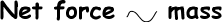We found two relations of force. Now it is time to combine them.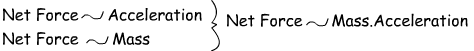Force is linearly proportional to the mass and acceleration. If the mass is constant, when we increase the force the object gains acceleration with the same amount or, if the force is constant, when we decrease the mass acceleration increases with the same amount.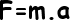ExampleWhere, F is the force and its unit is Newton, m is mass and has the unit kg and a is the acceleration has unit m/s².

Find the acceleration of the block given in the picture below.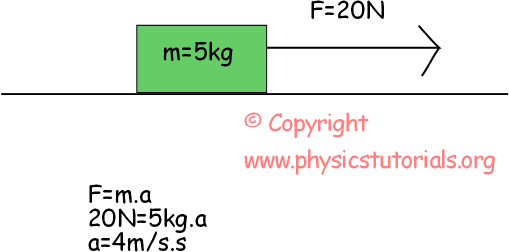How can we find the direction of acceleration? It is a vector quantity, so it must have a direction beside its magnitude. Look at the above example, mass is a scalar quantity, force is a vector quantity. So, net force is the only quantity which determines the direction of acceleration. For this example, our acceleration is 4m/s² to the rightward.

Example Given in the picture below, a horse is pulling the horsebox having 8 kg mass in it with a force of 40N; if the applied force has an angle of 37º to the horizontal; calculate the acceleration of the horsebox.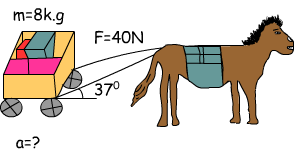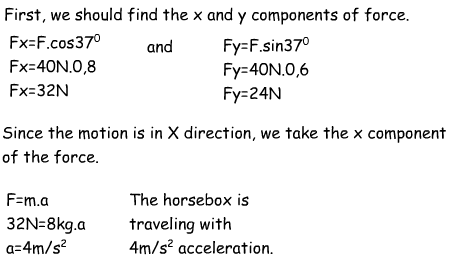Direction of the acceleration is in the direction of Fx which is the net force in direction of motion.

Mass and Weight

Mass and weight are the most confusing concepts in physics. Sometimes we use them interchangeably in daily life; however, in physics we must be careful while using them. Mass is the quantity of matter. As given in the definition it contains only the magnitude thus we say that it is a scalar quantity. Mass is constant everywhere. Weight is of course related to the mass, but, it has a little bit different definition. Weight is the force of gravity acting upon the things. Since it is a kind of force, weight is a vector quantity. Weight of the object can be different in different part of the world. Since it is linearly proportional to gravitation, it changes when the value of gravitation changes. How can we find the weight of the object? We say that it is a force of gravity; we calculate the weight as given below;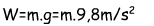Where, m is the mass and g is the gravitational acceleration.

Example Find the weight of the object having mass 15 kg.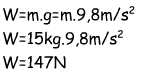Dynamics Exams

Related